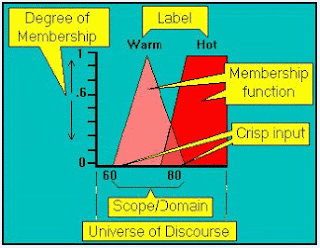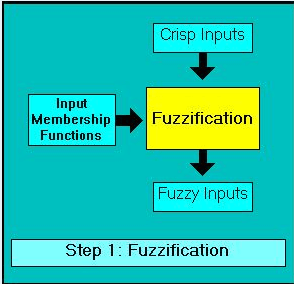Wednesday, December 19, 2012

The Basic Concepts of Fuzzy LogicFigure 1. Boolean Logic vs Fuzzy Logic Difference Illustration

Robometricschool. - Lots of control systems at the moment who want the output to have high accuracy, so that the control system requires a fairly complex system. With the fuzzy logic system then we do not need a very complex control system.

Fuzzy logic system first discovered by Prof.. Lotfi Zadeh in the mid-1960s at the University of California. This system was created for Boolean logic does not have high precision, only to have a logic 0 and 1 only. So as to make the system has a high accuracy, we can not use the Boolean logic.

Furthermore, Prof.. Lotfi Zadeh Fuzzy Logic says that integration into information systems and engineering processes are generating applications such as control systems, household appliance, and system decision makers more flexible, stable, and powerful than conventional systems. In this case we can say that the fuzzy logic engine lead in the development of higher intelligence (machine Intelligency Quotient / MIQ).

Fuzzy Logic is a rise of Boolean logic dealing with the concept of partial truth. When classical logic to claim that everything can be expressed in terms of binary (0 or 1, black or white, yes or no), fuzzy logic replaces Boolean truth with a level of truth. As an illustration to tell the difference between a Boolean fuzzy logic as shown in figure 1 above.

From the example of figure 1 above, we know that when the temperature was at 75 ° so that the system will first be confused as boundary conditions for cold <75 and heat conditions> 75 °, in fuzzy logic, a temperature of 75 ° can be expressed with 00:50 and 12:50 hot winter. 0:50 Taking the value derived from this process will be explained in fuzzification process.

To facilitate the basic concepts of fuzzy logic, it can be described by the following figure 2 bellow:Figure 2. Basic Concepts in Fuzzy Logic System Diagram

• Degree of membership
The function of the degree of membership is to give weight to the input we have given, so that input can be expressed in value earlier. Also be interpreted as the degree to which the values ​​compatible with crisp membership function (from 0 to 1), also referred to as the level of membership, the truth value, or input fuzzy instance temperature is cold, with the degree of membership that cold temperatures can have a value eg 0 , 5. Limit of degree of membership is from 0-1.

• Scope / Domain
Scope / Domain is the width of the membership function or a boundary of a particular set of inputs. The range of concepts, usually numbers, where membership functions mapped. For example, cold temperatures are from 10-50 degrees, very quickly is from 200-500 rpm.

• Label
A label is a descriptive name used to identify the membership function. It can also be interpreted as a word - the word to give a statement on the Scope / Domain. For example: heat, cold, fast, very fast, etc.

• Membership Function
A that represents the shape of a boundary of scope / domain. In other words that define the fuzzy membership functions are set with crisp mapping input from domain to degrees of membership.

• Crisp Input
It is firm and certain inputs such as analog input value that we provide to find degree of membership.

• Universe of discourse
Limit input we have given in designing a fuzzy system. This limit is different from the limit of scope / domain. Universe of discourse is the limit all the inputs to be provided while the scope / domain is a boundary that determines that the input is expressed heat, cold, fast, etc.

In the fuzzy logic system, there are three kinds of processes are carried out, namely Fuzzification, Rule evaluation and Defuzification. Here's an explanation of each process.
• Fuzzification
This process serves to change the scale analog input fuzzy. Fuzzification means changing inputs are definite truth value (crisp input) into the form of fuzzy input. Please view figure 3 bellow:Figure 3. Fuzzification Process

• Membership Function
This process serves to to look for a value of fuzzy input fuzzy output.The process is as follows: a fuzzy input values ​​from the fuzzification process and then inserted into a rule that was created to serve as a fuzzy output. Please view figure 4 bellow:Figure 4. Rule Evaluation Process

• Defuzzicication
Defuzzification means changing the fuzzy output into crisp value based on predetermined membership functions. This process serves to determine a crisp output value.Figure 5. Defuzzification Process

There are many defuzzification methods that have been successfully applied to a wide range of issues, here discussed five of these methods, namely:
1. Centroid method.
2. Height method.
3. First (or Last) of Maxima
4. Mean-Max method
5. Weighted Average
Before defuzzification, to do the composition, the aggregate results of all clipping fuzzy rules to obtain a single fuzzy set.